# Three forces acting on an object are given by F1 = (-2.00 i + 2.00 j) N, and F2 = (5.00 i – 3.00j) N, and F3 = (- 45.0 i) N. The object experiences an acceleration of magnitude 3.75 m/s2. (a)What is the direction of the acceleration? (b) What is the mass of the object? (c) If the object isinitially at rest, what is its speed after 10.0 s? (d) What are the velocitycomponents of the object after 10.0 s?

Question
62 views

Three forces acting on an object are given by F1 = (-2.00 i + 2.00 j) N, and F2 = (5.00 i – 3.00
j) N, and F3 = (- 45.0 i) N. The object experiences an acceleration of magnitude 3.75 m/s2
. (a)
What is the direction of the acceleration? (b) What is the mass of the object? (c) If the object is
initially at rest, what is its speed after 10.0 s? (d) What are the velocity
components of the object after 10.0 s?

check_circle

Step 1

Hello. Since your question has multiple sub-parts, we will solve the first three sub-parts for you. If you want remaining sub-parts to be solved, then please resubmit the whole question and specify those sub-parts you want us to solve.

Given vector forces are,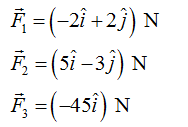Given acceleration is,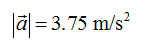Step 2

Consider m as the mass of the object. The net force acting on the mass m is,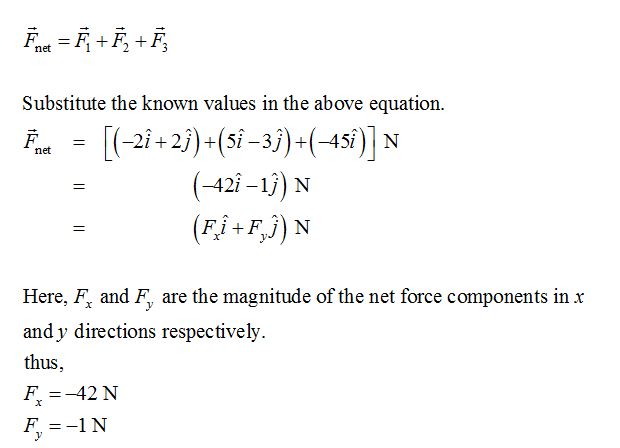Since both Fx and Fy are negative, the net force lies in the third quadrant.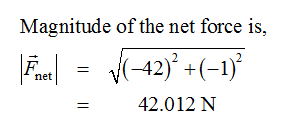Step 3

(a)

The acceleration of the body will be in the direction of the net force acting on the body.

The direction of the force can be defined in terms of angular displacement anticlockwise with respect to the x-axis.

And it can be calculated as,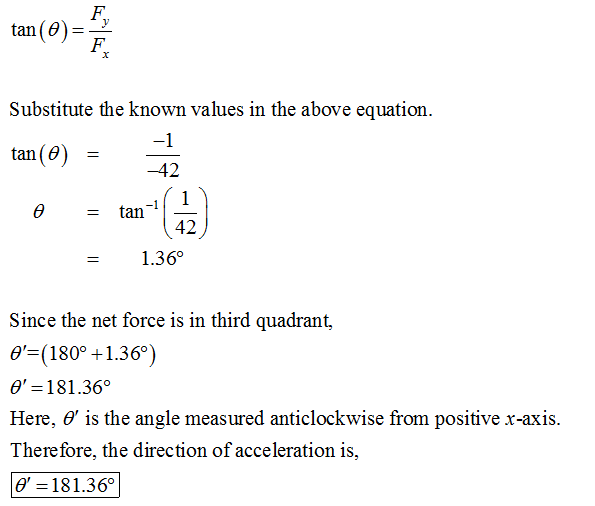...

### Want to see the full answer?

See Solution

#### Want to see this answer and more?

Solutions are written by subject experts who are available 24/7. Questions are typically answered within 1 hour.*

See Solution
*Response times may vary by subject and question.
Tagged in